# 函数的定义

return[表达式]结束函数，选择性地返回一个值给调用方。不带表达式的return相当于返回None。

def fib(n):
a,b=0,1
while a<n:
print(a,end=" ")
a,b=b,a+b
print()
fib(2000)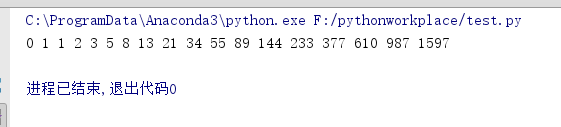print(fib)
f=fib
f(
100)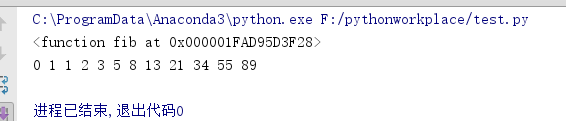print(fib(0))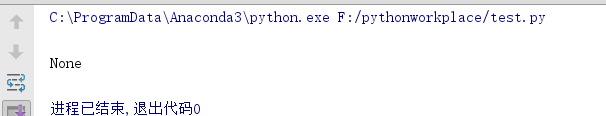print(fib(1))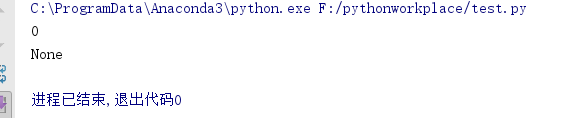def fib2(n):
result=[]
a,b=0,1;
while a<n:
result.append(a)
a,b=b,a+b
return result
f100=fib2(100)
print(f100)# 返回值与返回值类型

def fun1():
pass
print(fun1())
def fun2():
return '123'
print(fun2())
def fun_list():
return ['123','xyz',456]
print(fun_list())
def fun_tuple1():
return 1,2,3
print(fun_tuple1())
def fun_tuple2():
return (1,2,3)
print(fun_tuple2())

None

123

['123', 'xyz', 456]

(1, 2, 3)

(1, 2, 3)

fun1()返回None，fun2()的返回值类型是字符串，fun_list()、fun_tuple1()、fun_tuple2()的返回值大于1个，fun_list()返回的是一个列表，fun_tuple1()、fun_tuple2()等价，都是一个元组。

def fun_tuple():
return 'abc',5,['k','p']
aTuple=fun_tuple()
x,y,z=fun_tuple()
(a,b,c)=fun_tuple()
print(aTuple)
print(x,y,z)
print((a,b,c))

('abc', 5, ['k', 'p'])

abc 5 ['k', 'p']

('abc', 5, ['k', 'p'])

def foo():
print(
'1')
bar()

def bar():
print(
'2')
foo()

1

2

# 内部/内嵌函数

def foo():
print('1')
def bar():
print('2')
bar()
foo()

1

2

# 传递函数

1. 被引用（访问或者以其他变量作为其别名）
2. 作为参数传入函数
3. 作为字典、列表等容器对象的元素

def foo():
print('1')
bar=foo
bar()

1

def foo():
print('1')
def bar(argfunc):
argfunc()
bar(foo)

1

# 参数的种类

## 位置参数

def info(name,sex,grades,school='abc'):
print(name)
print(sex)
print(school)
info('Jennifer','female','3')
print()
print()
info(grades='1',sex='male',name='frank')

Jennifer

female

3

abc

Jennifer

female

1

lalala

frank

male

1

abc

## 参数默认值

def ask_ok(prompt,retries=4,reminder='Please try again!'):
while True:
ok=input(prompt)
if ok in ('y','ye','yes'):
return True
if ok in ('n','no','nop','nope'):
return False
retries=retries-1
if retries<0:
raise ValueError('invalid user response')
print(reminder)

只给出必需的参数：ask_ok('Do you really want to quit?')

给出一个可选的参数：ask_ok('OK to overwrite the file?', 2)

i=5
def f(arg=i):
print(arg)
i=6
f()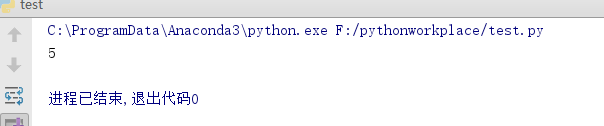def f(a,L=[]):
L.append(a)
return L
print(f(1))
print(f(2))
print(f(3))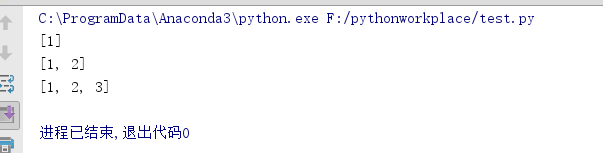def f(a,L=None):
if L is None:
L=[]
L.append(a)
return L
print(f(1))
print(f(2))
print(f(3))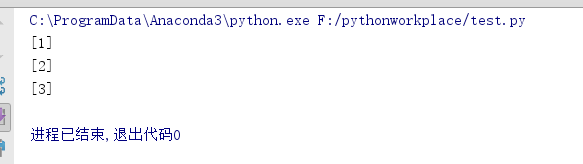## 命名关键字参数

def temp(a,b,c):

print(a)

print(b)

print(c)

temp(1,3,c=99)

temp(a=1,b=2,c=3)

temp(1,b=2,c=100)

temp(100,b=200,c=300)

temp(a=100,32,100) #错误语法，关键字参数不能在普通参数的前面

hi = {"a":100,"b":99,"c":1000}

temp(**hi) #等同于下面#

temp(a=100,b=99,c=1000)

temp(c=100,b=38,a=10)

def parrot(voltage,state='a stiff',action='voom',type='Norwegian Blue'):
print("--This parrot wouldn't",action,end=' ')
print("if you put",voltage,"volts though it.")
print("-- Lovely plumage, the", type)
print("-- It's", state, "!")

• 一个位置参数：parrot(1000)
• 一个关键字参数：parrot(voltage=1000)
• 两个关键字参数;parrot(voltage=1000000, action='VOOOOOM')
• 两个关键字参数;parrot(action='VOOOOOM', voltage=1000000)
• 三个位置参数：parrot('a million', 'bereft of life', 'jump')
• 一个位置参数，一个关键字参数：parrot('a thousand', state='pushing up the daisies')

• 丢失必要参数：parrot()
• 同一参数的重复值 ：parrot(110, voltage=220)
• 未知的关键字参数：parrot(actor='John Cleese')

def function(a):
pass
function(0,a=0)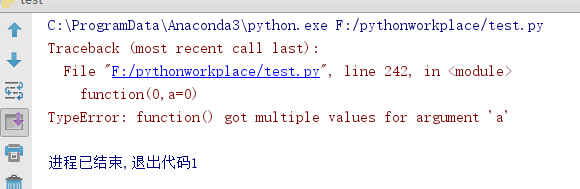## 关键字参数：**others，便于函数功能的扩展

def cheeseshop(kind, *arguments, **keywords):
print("-- Do you have any", kind, "?")
print("-- I'm sorry, we're all out of", kind)
for arg in arguments:
print(arg)
print("-" * 40)
for kw in keywords:
print(kw, ":", keywords[kw])

cheeseshop("Limburger", "It's very runny, sir.",
"It's really very, VERY runny, sir.",
shopkeeper="Michael Palin",
client="John Cleese",
sketch="Cheese Shop Sketch")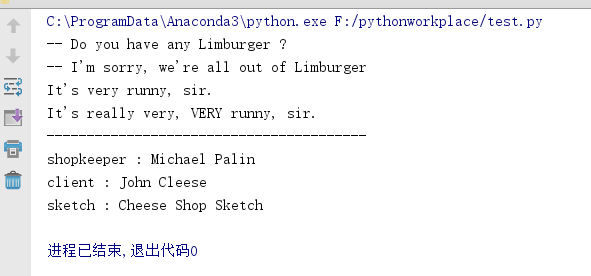## 任意的参数列表 *others

def write_multiple_items(file, separator, *args):
file.write(separator.join(args))

def concat(*args, sep="/"):

return sep.join(args)
print(concat("earth", "mars", "venus"))
print(concat("earth", "mars", "venus", sep="."))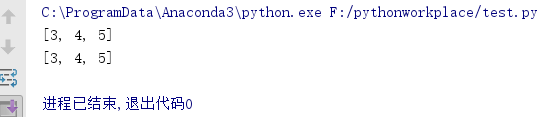## 解包参数列表

list(range(3,6))
print(list(range(3,6)))
args=[3,6]
print(list(range(*args)))def parrot(voltage, state='a stiff', action='voom'):
print("-- This parrot wouldn't", action, end=' ')
print("if you put", voltage, "volts through it.", end=' ')
print("E's", state, "!")
d = {"voltage": "four million", "state": "bleedin' demised", "action": "VOOM"}
parrot(**d)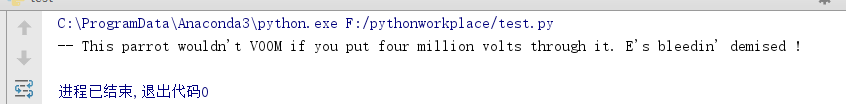有问题请下方评论，转载请注明出处，并附有原文链接，谢谢！如有侵权，请及时联系。

11-073241
11-17
12-0942
08-041920
07-151万+
05-233353
06-27858
03-161448
06-017065
03-306371
02-03285
08-052万+
02-20809
11-2761
03-12358
04-061500
01-27267
10-064114

### “相关推荐”对你有帮助么？

•非常没帮助
•没帮助
•一般
•有帮助
•非常有帮助被折叠的  条评论 为什么被折叠?到【灌水乐园】发言WXiujie123456

¥2 ¥4 ¥6 ¥10 ¥20余额支付 (余额：-- )扫码支付获取中扫码支付点击重新获取扫码支付1.余额是钱包充值的虚拟货币，按照1:1的比例进行支付金额的抵扣。
2.余额无法直接购买下载，可以购买VIP、C币套餐、付费专栏及课程。余额充值